# Algebra - math word problems

#### Number of problems found: 2671

• The difference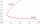The difference of two numbers is 1375. If their exact quotient is 12. Find the two numbers
• Iron pole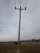The iron pole is in the ground 2/5 of its length, partly above the ground 1/3 is yellow, and the unpainted section is 6 m long. How long is the entire column?
• Loan 5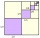Abdul takes a loan of 200000 from Ali and agrees to repay in number of instalment, each instalment begin with the 2nd exceeding the previous one by 1000, if the first instalment is 500, find how many instalment will be necessary to be wipe out the loan? C
• Dance groupThe dance group formed groups of 4, 5, and 6 members. Always one dancer remains. How many dancers were there in the whole group?
• Water tankWhat is the height of the cuboid-shaped tank with the bottom dimensions of 80 cm and 50 cm if the 480 liters of water reaches 10 cm below the top?
• Last pageTwo consecutive sheets dropped out of the book. The sum of the numbers on the sides of the dropped sheets is 154. What is the number of the last page of the dropped sheets?
• Four paversFour pavers would pave the square in 18 days. How many pavers do you need to add to done work in 12 days?
• Centroid - two bodiesA body is composed of a 0.8 m long bar and a sphere with a radius of 0.1m attached so that its center lies on the longitudinal axis of the bar. Both bodies are of the same uniform material. The sphere is twice as heavy as the bar. Find the center of gravi
• Shopping center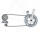The shopping center buys from the manufacturer bikes at a purchase price of 180 €. It sells them for a sale price of 250 €. However, in the advertising for the sale of these goods, the shopping center spent 20% of the selling price of all bicycles in stoc
• PotatoesFor three days the store sold 1400 kg of potatoes. The first day they sold 100 kilograms of potatoes less than the second day, the third-day three-fifths of what they sold the first day. How many kgs of potatoes sold every day?
• Nine booksNine books are to be bought by a student. Art books cost \$6.00 each and biology books cost \$6.50 each . If the total amount spent was \$56.00, how many of each book was bought?
• Sand castle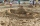Tim and Tom built a sand castle and embellished it with a flag. Half the pole with the flag plunged into the castle. The highest point of the pole was 80 cm above the ground, its lowest point 20 cm above the ground. How high was the sand castle?
• The sides 2The sides of a trapezoid are in the ratio 2:5:8:5. The trapezoid’s area is 245. Find the height and the perimeter of the trapezoid.
• Find parametersFind parameters of the circle in the plane - coordinates of center and radius: ?The school received money from the sponsor. She used one-third to buy computers, half of the rest to adjust the gym. It still has 4000 euros left. How many euros did the sponsor donate to the school?
• The workThe work was to be done by 150 workers. At the beginning of their work, their number reduced by 40, which increased the time of work by 5 and 1/3 of the schedule. How long did work take?
• Small painters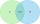There are ten pupils in the painting group. Eight pupils paint with watercolors and nine pupils with tempera colors. How many pupils paint both water and tempera colors when each pupil paints?
• Three peopleThree people start doing a work at a same time. The first worked only 2 hours. The second ended 3 hours before the end. On an individual basis, it would take the first time to do the work 10 hours, second 12 hours and 15 hours third. How many hours did it
• Find the 9Find the missing angle in the triangle and then name triangle. Angles are: 95, 2x+15, x+3
• Profit growthThe profit of a company increased by 25% during the year 1992, increased by 40% during the year 1993, decreased by 20% in the year 1994 and increased by 10% during the year 1995. Find the average growth in the profit level over the four years periods?

Do you have an interesting mathematical word problem that you can't solve it? Submit a math problem, and we can try to solve it.

We will send a solution to your e-mail address. Solved examples are also published here. Please enter the e-mail correctly and check whether you don't have a full mailbox.

Please do not submit problems from current active competitions such as Mathematical Olympiad, correspondence seminars etc...